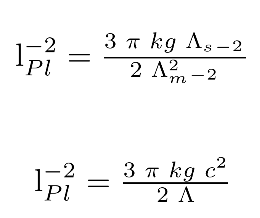# Hi, I have a dimensional problem

## Recommended Posts

lPl-2 is the vacuum energy from QFT in m-2

on the right I have 1(kg s-2) or 1(N/m) inverse of a surface tension.

Is my problem insolvent or do you see a solution please?

Edited by stephaneww
##### Share on other sites

Why doesn't it have units of energy?

Can you show where you got this equation?

##### Share on other sites

18 minutes ago, swansont said:

Why doesn't it have units of energy?

Uh m-2 is the dimension of the cosmological constant.

for lPl-2 of the QFT I have only one reference in French : https://www.unige.ch/communication/communiques/2019/cosmologie-une-solution-a-la-pire-prediction-en-physique/

18 minutes ago, swansont said:

Can you show where you got this equation?

This equality comes from a speculation published in French on my blog. I can't put in latex a clean way to reach  the equality that I have a problem with here.

Edited by stephaneww
##### Share on other sites

1 hour ago, stephaneww said:

Uh m-2 is the dimension of the cosmological constant.

for lPl-2 of the QFT I have only one reference in French : https://www.unige.ch/communication/communiques/2019/cosmologie-une-solution-a-la-pire-prediction-en-physique/

The cosmological constant energy density "units" are for when you set G = c = 1, so you can't really distinguish between the two uses of the cosmological constant (and is the answer to why it doesn't have units of energy)

Is that also happening for your other equation? i.e. was it from an equation with normal units, or one where they were ignored?

Since you're asking this without providing context, it's very difficult to see if this is the problem

The cosmological constant has the same effect as an intrinsic energy density of the vacuum, ρvac (and an associated pressure). In this context, it is commonly moved onto the right-hand side of the equation, and defined with a proportionality factor of 8π: Λ = 8 π ρvac , where unit conventions of general relativity are used (otherwise factors of G and c would also appear, i.e., Λ = 8 π ρvac G / c4 = κ ρvac , where κ is Einstein's rescaled version of the gravitational constant G). It is common to quote values of energy density directly, though still using the name "cosmological constant", using Planck units so that 8πG = 1. The true dimension of Λ is length−2.

IOW, Λ =  κ ρvac and you call it an energy density by assuming  κ is 1. But it's not actually 1

Quote

This equality comes from a speculation published in French on my blog. I can't put in latex a clean way to reach  the equality that I have a problem with here.

I meant "where in standard physics literature did this equation come from"

You need to have started with mainstream physics at some point.

##### Share on other sites

2 hours ago, swansont said:

Is that also happening for your other equation? i.e. was it from an equation with normal units, or one where they were ignored?

no, precisely I take into account the dimensions: originally I have an equality which in a form is in Joules and in a second form it gives a numerical value close to the value of Λ in m-2. I justify this difference because of a difference in degrees of freedom.

2 hours ago, swansont said:

Since you're asking this without providing context, it's very difficult to see if this is the problem

edit :

I tried to explain the process clearly but I can't. I'll put it off for another day.

2 hours ago, swansont said:

IOW, Λ =  κ ρvac and you call it an energy density by assuming  κ is 1. But it's not actually 1

I never use this : for me ρvac = Λ FPl /8 pi   and  ρvac from QFT = lPl2   FPl

(FPl =c4/G)

2 hours ago, swansont said:

I meant "where in standard physics literature did this equation come from"

You need to have started with mainstream physics at some point.

it is not in the standard physics. it comes from my model derived from the ΛCDM model and which approaches it under a new angle. but I find the essential of the results of the standard model. (mass at the Hubble radius and critical energy density of the universe in a certain way). other points are still speculative including this one

Edited by stephaneww
##### Share on other sites

.not to another day but later

5 hours ago, stephaneww said:

lPl-2 is the vacuum energy from QFT in m-2

on the right I have (kg s-2) or1(N/m) inverse of a surface tension.

Is my problem insolvent or do you see a solution please?

and

remplace s-2 et m2 in Joules  kg m2 s-2 by Λs-2 and Λm-2

correction :## Create an account

Register a new account